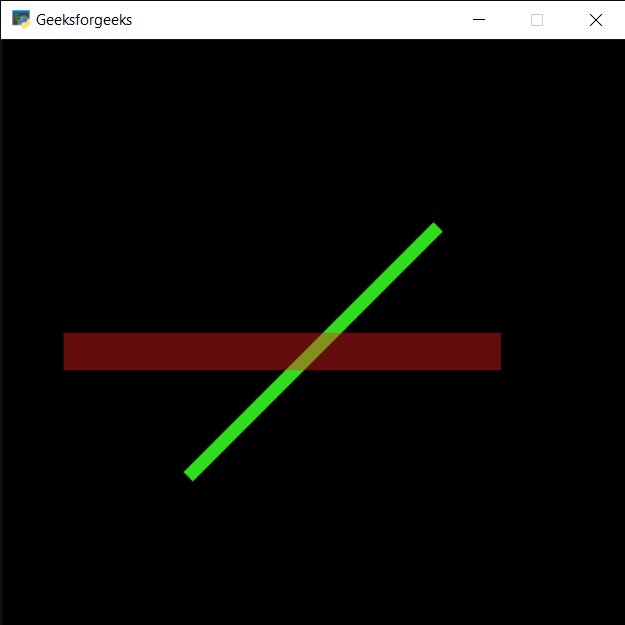Open In App

# PYGLET – Drawing Line

In this article, we will see how we can draw line on window in PYGLET module in python. Pyglet is easy to use but powerful library for developing visually rich GUI applications like games, multimedia etc. A window is a “heavyweight” object occupying operating system resources. Windows may appear as floating regions or can be set to fill an entire screen (fullscreen). Spectral line shape describes the form of a feature, observed in spectroscopy, corresponding to an energy change in an atom, molecule or ion. Ideal line shapes include Lorentzian, Gaussian and Voigt functions, whose parameters are the line position, maximum height and half-width. Line is drawn with the help of shapes module in pyglet.

We can create a window with the help of command given below

```# creating a window
window = pyglet.window.Window(width, height, title)```

In order to create window we use Line method with pyglet.shapes
Syntax : shapes.Line(co_x1, co_y1, co_x2, co_y2, width, color = (50, 225, 30), batch=batch)
Argument : It takes starting and end position in form of pair of two integers, width of line, color of line and last is batch object
Return : It returns Line object

Below is the implementation

## Python3

 `# importing pyglet module``import` `pyglet` `# importing shapes from the pyglet``from` `pyglet ``import` `shapes` `# width of window``width ``=` `500``  ` `# height of window``height ``=` `500``  ` `# caption i.e title of the window``title ``=` `"Geeksforgeeks"``  ` `# creating a window``window ``=` `pyglet.window.Window(width, height, title)` `# creating a batch object``batch ``=` `pyglet.graphics.Batch()`  `# properties of line``# first co-ordinates of line``co_x1 ``=` `150``co_y1 ``=` `150` `# second co-ordinates of line``co_x2 ``=` `350``co_y2 ``=` `350` `# width of line``width ``=` `10` `# color = green``color ``=` `(``50``, ``225``, ``30``)` `# creating a line``line1 ``=` `shapes.Line(co_x1, co_y1, co_x2, co_y2, width, color ``=` `(``50``, ``225``, ``30``), batch ``=` `batch)` `# changing opacity of the line1``# opacity is visibility (0 = invisible, 255 means visible)``line1.opacity ``=` `250`  `# creating another line with properties``# x1, y1 = 50, 250``# x2, y2 = 400, 250``# color = red``line2 ``=` `shapes.Line(``50``, ``250``, ``400``, ``250``, ``30``, color ``=` `(``250``, ``30``, ``30``), batch ``=` `batch)` `# changing opacity of the line2``# opacity is visibility (0 = invisible, 255 means visible)``line2.opacity ``=` `100`  `# window draw event``@window``.event``def` `on_draw():``    ` `    ``# clear the window``    ``window.clear()``    ` `    ``# draw the batch``    ``batch.draw()` `# run the pyglet application``pyglet.app.run()`

Output :